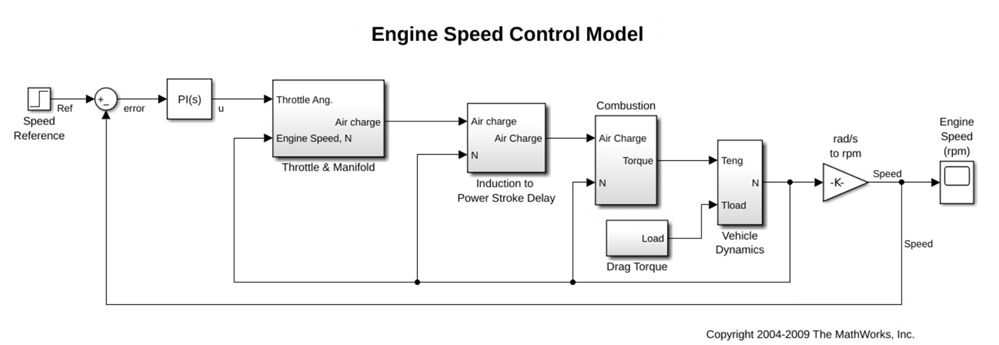# What Is a Block Diagram?

A block diagram consists of blocks that represent different parts of a system and signal lines that define the relationship between the blocks. Block diagrams are used in engineering areas such as feedback control theory and signal processing. A block within a block diagram may define a function, an operation, or a model in itself.Block diagram representation.

## Use Block Diagrams to Graphically Represent Dynamic Systems

Block diagrams are widely used by engineers for controls, signal processing, communications, and mechatronics. Engineers build and use block diagrams to:

• Provide a high-level graphical representation for a mathematical model of real-world systems, as in the figure below.
• Understand the relationship and interdependencies among different parts of a system, such as a house heating system.
• Analyze dynamic system behavior in time and frequency domains.

Engineers rely on the Simulink® environment to efficiently build and simulate block diagrams for multidomain systems. This environment provides:

• A graphical editor for building and managing hierarchical block diagrams
• An extensive set of block libraries and templates to design block diagrams
• Solvers to simulate a range of systems, including continuous-time (analog), discrete-time (digital), hybrid (mixed-signal), discrete-event, and multirate systems

For details on modeling and simulating block diagrams, see Simulink.